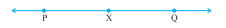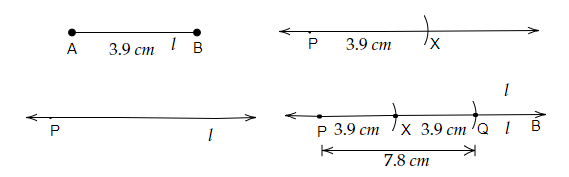(Hint : Construct $\overline{\mathrm{PX}}$ such that length of $\overline{\mathrm{PX}}=$ length of $\overline{\mathrm{AB}}$; then cut off $\overline{\mathrm{XQ}}$ such that $\overline{\mathrm{XQ}}$ also has the length of $\overline{\mathrm{AB}}$.)"
">

# Given $\overline{\mathrm{AB}}$ of length $3.9 \mathrm{~cm}$, construct $\overline{\mathrm{PQ}}$ such that the length of $\overline{\mathrm{PQ}}$ is twice that of $\overline{\mathrm{AB}}$. Verify by measurement.(Hint : Construct $\overline{\mathrm{PX}}$ such that length of $\overline{\mathrm{PX}}=$ length of $\overline{\mathrm{AB}}$; then cut off $\overline{\mathrm{XQ}}$ such that $\overline{\mathrm{XQ}}$ also has the length of $\overline{\mathrm{AB}}$.)"

Given:

$\overline{AB}$ of length $3.9\ cm$.

To do:

We have to construct $\overline{PQ}$ that is twice that of $\overline{AB}$ and verify by measurement.

Solution:Steps of construction:

(i) First, let us draw a line 'l' and mark a point P on the line 'l'.

(ii) Now, by taking the length of $\overline{AB}$ with compasses let us draw an arc on the line 'l' and point it as X, by pointing the pointer of the compasses at point P.

(iii) With the same measure by pointing the pointer of compasses at point X, draw another arc cutting the line 'l' and point it as Q.

(iv) Therefore, the line segment PQ which is twice the length of $\overline{AB}$ is formed.

(v) We can verify the measurement by placing the ruler's starting point on point P and measuring it to point Q.

(vi) Which measures $7.8 \cm$ i.e., twice the length of $\overline{AB}$.

Updated on: 10-Oct-2022

29 Views